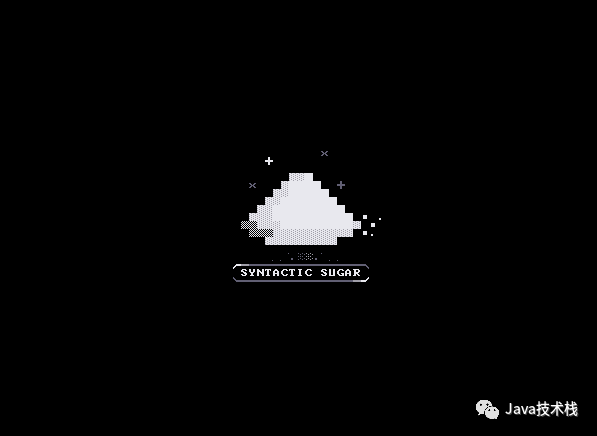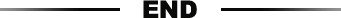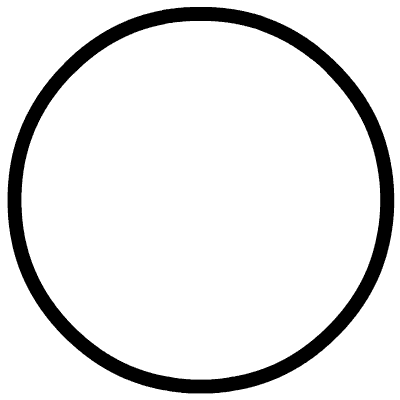Java作為一種與平臺無關的高級語言，當然也含有語法糖，這些語法糖並不被虛擬機所支持，在編譯成字節碼階段就自動轉換成簡單常用語法。

1. 泛型與類型擦除

2. 自動裝箱與拆箱

3. 變長參數

4. 增強for循環

5. 內部類

6. 枚舉類

Java語言並不是一開始就支持泛型的。在早期的JDK中，只能通過Object類是所有類型的父類和強制類型轉換來實現泛型的功能。強制類型轉換的缺點就是把編譯期間的問題延遲到運行時，JVM並不能為我們提供編譯期間的檢查。

``/**``* 在源代碼中存在泛型``*/``public static void main(String[] args) {``    Map<String,String> map = new HashMap<String,String>();``    map.put("hello","你好");``    String hello = map.get("hello");``    System.out.println(hello);``}``

``public static void main(String[] args) {``    HashMap map = new HashMap(); //類型擦除``    map.put("hello", "你好");``    String hello = (String)map.get("hello");//強制轉換``    System.out.println(hello);``}``

Java中的自動裝箱與拆箱指的是基本數據類型與他們的包裝類型之間的相互轉換。

``public static void main(String[] args) {``    Integer a = 1;``    int b = 2;``    int c = a + b;``    System.out.println(c);``}``

``public static void main(String[] args) {``    Integer a = Integer.valueOf(1); // 自動裝箱``    byte b = 2;``    int c = a.intValue() + b;//自動拆箱``    System.out.println(c);``}``

``public class Varargs {``    public static void print(String... args) {``        for(String str : args){``            System.out.println(str);``        }``    }``    public static void main(String[] args) {``        print("hello", "world");``    }``}``

``public class Varargs {``    public Varargs() {``    }``    public static void print(String... args) {``        String[] var1 = args;``        int var2 = args.length;``        //增強for循環的數組實現方式``        for(int var3 = 0; var3 < var2; ++var3) {``            String str = var1[var3];``            System.out.println(str);``        }``    }``    public static void main(String[] args) {``        //變長參數轉換為數組``        print(new String[]{"hello", "world"});``    }``}``

``public static void main(String[] args) {``    String[] params = new String[]{"hello","world"};``    //增強for循環對象為數組``    for(String str : params){``        System.out.println(str);``    }``    List<String> lists = Arrays.asList("hello","world");``    //增強for循環對象實現Iterable接口``    for(String str : lists){``        System.out.println(str);``    }``}``

``public static void main(String[] args) {``   String[] params = new String[]{"hello", "world"};``   String[] lists = params;``   int var3 = params.length;``   //數組形式的增強for退化為普通for``   for(int str = 0; str < var3; ++str) {``       String str1 = lists[str];``       System.out.println(str1);``   }``   List var6 = Arrays.asList(new String[]{"hello", "world"});``   Iterator var7 = var6.iterator();``   //實現Iterable接口的增強for使用iterator接口進行遍歷``   while(var7.hasNext()) {``       String var8 = (String)var7.next();``       System.out.println(var8);``   }``}``

``public class Outer {``    class Inner{``    }``}``

``class Outer\$Inner {``    Outer\$Inner(Outer var1) {``        this.this\$0 = var1;``    }``}``

``public enum Fruit {``    APPLE,ORINGE``}``

``//繼承java.lang.Enum並聲明為final``public final class Fruit extends Enum``{``    public static Fruit[] values()``    {``        return (Fruit[])\$VALUES.clone();``    }``    public static Fruit valueOf(String s)``    {``        return (Fruit)Enum.valueOf(Fruit, s);``    }``    private Fruit(String s, int i)``    {``        super(s, i);``    }``    //枚舉類型常量``    public static final Fruit APPLE;``    public static final Fruit ORANGE;``    private static final Fruit \$VALUES[];//使用數組進行維護``    static``    {``        APPLE = new Fruit("APPLE", 0);``        ORANGE = new Fruit("ORANGE", 1);``        \$VALUES = (new Fruit[] {``            APPLE, ORANGE``        });``    }``}``

Java遠不止這些語法糖，JDK7、JDK8添加的新特性都有很多高級的語法糖。https://www.wxwenku.com/d/109858453